#### Parameter Estimates

When the fit process is complete, the VARIOGRAM procedure produces the Parameter Estimates table with information about the fitted model parameters. The table includes estimates of the parameters, their approximate standard error, the statistical degrees of freedom DF, the corresponding t statistic, and its approximate p-value. For a model with q parameters that fits an empirical semivariogram of k nonmissing lags,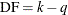.

Note: Parameter estimates might have nonzero standard errors even in the rather extreme case where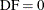. This can typically occur when there are active optimization constraints in the fitting process.

You can request the confidence intervals for the parameter estimates of a fitted model by specifying the CL option of the MODEL statement. These confidence intervals are computed using the Wald-based formula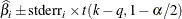where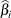is the ith parameter estimate,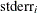is its estimated approximate standard error,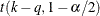is the t statistic withdegrees of freedom. The confidence intervals are only asymptotically valid. The significance levelused in the construction of these confidence limits can be set with the ALPHA= option of the MODEL statement; the default value is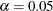.

Specify the COVB and the CORRB options in the MODEL statement to request the approximate covariance and approximate correlation matrices of the fitted parameters, respectively. These matrices are based on the optimization process results. In agreement with reporting similar optimization output in SAS/STAT software, parameters with active restraints have zeros in the corresponding rows and columns in the covariance and correlation matrices, and display 1 in the correlation matrix diagonal.